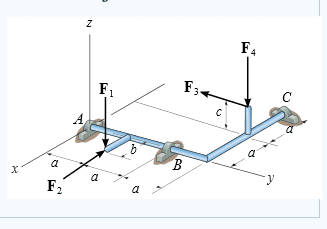# 3D Rigid Body Equilibrium

## Homework Statement

The rod assembly shown has a ball-and-socket joint at A and a smooth journal bearing at C. The forces F1=600N , F2=410N , F3=460N , and F4=950N are applied as shown in the figure. The geometry of the rod assembly is given as a=0.900m , b=0.650m , and c=0.800m. Neglect the weight of the rod. The journal bearing supports the rod in such a manner that it is free to rotate about the y axis.

1.)Determine the magnitude of the y component of the reaction at C

2.) Determine the magnitude of the z component of the reaction exerted on the rod at C.

3.) Determine the magnitude of the z component of the reaction on the rod at B.

4.) Determine the magnitude of the x component of the reaction on the rod at B.

5.) Determine the magnitude of the z component of the reaction on the rod at A

6.) Determine the magnitude of the x component of the reaction on the rod at A